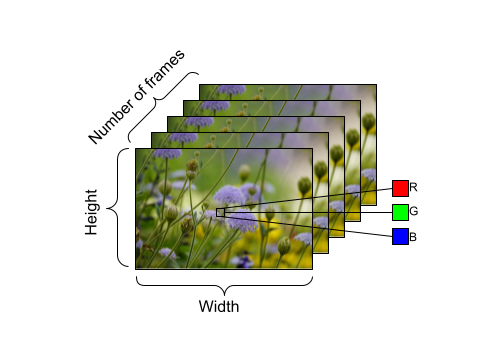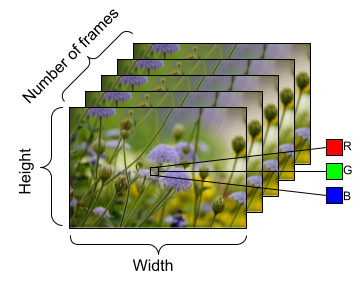codelab · deep learning ·Using TensorFlow for Deep Learning on Video Data
January 19, 2023Posted by Shilpa Kancharla

Video data contains a rich amount of information, and has a more complex and large structure than image data. Being able to classify videos in a memory-efficient way using deep learning can help us better understand the contents within the data. On tensorflow.org, we have published a series of tutorials on how to load, preprocess, and classify video data. Here are quick links to each of these tutorials:

In this blog post, we thought it would be interesting to go more in depth about certain parts of some tutorials, and talk about how you can incorporate these parts to build your own models that can process video or three-dimensional data (such as MRI scans) in a memory-efficient manner using TensorFlow, such as leveraging Python generators and resizing, or downsampling, the data.Example of shape of video data, with the following dimensions:number of frames (time) x height x width x channels.

## FrameGenerator to load video data

From the Load video data tutorial, let’s take the opportunity to talk about the main workhorse of the majority of these tutorials: the FrameGenerator class. Through this class, we are able to yield the tensor representation of the video and the label, or class, of the video.

 class FrameGenerator:  def __init__(self, path, n_frames, training = False):    """ Returns a set of frames with their associated label.      Args:        path: Video file paths.        n_frames: Number of frames.        training: Boolean to determine if training dataset is being created.    """    self.path = path    self.n_frames = n_frames    self.training = training    self.class_names = sorted(set(p.name for p in self.path.iterdir() if p.is_dir()))    self.class_ids_for_name = dict((name, idx) for idx, name in enumerate(self.class_names))  def get_files_and_class_names(self):    video_paths = list(self.path.glob('*/*.avi'))    classes = [p.parent.name for p in video_paths]    return video_paths, classes  def __call__(self):    video_paths, classes = self.get_files_and_class_names()    pairs = list(zip(video_paths, classes))    if self.training:      random.shuffle(pairs)    for path, name in pairs:      video_frames = frames_from_video_file(path, self.n_frames)      label = self.class_ids_for_name[name] # Encode labels      yield video_frames, label

Upon creating the generator class, we use the function from_generator() to feed in the data to our deep learning models. Specifically, the from_generator() API will create a dataset whose contents are generated by a generator. Using Python generators can be more memory-efficient than storing an entire sequence of data in memory. Consider creating a generator class similar to FrameGenerator and using the from_generator() API to load data into your TensorFlow and Keras models.

output_signature = (tf.TensorSpec(shape = (None, None, None, 3),

dtype = tf.float32),
tf.TensorSpec(shape = (),

dtype = tf.int16))

train_ds = tf.data.Dataset.from_generator(FrameGenerator(subset_paths['train'],

10

training=True),

output_signature = output_signature)

## einops library for resizing video data

For the second tutorial on Video classification with a 3D convolutional neural network, let’s discuss the use of the einops library and how it can be incorporated into a Keras model backed by TensorFlow. This library is useful to perform flexible tensor operations and can be used with not only TensorFlow, but also JAX. Specifically in this tutorial, we use it to help with resizing the size of the data as it goes through the (2+1)D convolutional neural network we create. In the context of this second tutorial, we wanted to downsample the video data. Downsampling is particularly useful because it allows our model to examine specific parts of frames to detect patterns that may be specific to a certain feature in that video. Through downsampling, non-essential information can be discarded. It will allow for dimensionality reduction and therefore faster processing.

We use the functions parse_shape() and `rearrange()` from the `einops` library. The `parse_shape()` function used here maps the names of the axes to their corresponding lengths. It will return a dictionary containing this information, called `old_shape`. Next, we use the `rearrange()` function that allows you to reorder the axes for multidimensional tensors. Pass in the tensor, alongside the names of the axes you are trying to rearrange.

The notation `b t h w c -> (b t) h w c` here means we want to squeeze together the batch size (denoted by `b`) and time (denoted by `t`) dimensions to pass this data into the Keras `Resizing` layer object. When we instantiate the `ResizeVideo` class, we pass in the height and width values that we want to resize the frame to. Once this resizing is complete, we use the `rearrange()` function again to unsqueeze (using the notation `(b t) h w c -> b t h w c`) the batch size and time dimensions.

 class ResizeVideo(keras.layers.Layer):  def __init__(self, height, width):    super().__init__()    self.height = height    self.width = width    self.resizing_layer = layers.Resizing(self.height, self.width)  def call(self, video):    """      Use the einops library to resize the tensor.        Args:        video: Tensor representation of the video, in the form of a set of frames.      Return:        A downsampled size of the video according to the new height and width it should be resized to.    """    # b stands for batch size, t stands for time, h stands for height,    # w stands for width, and c stands for the number of channels.    old_shape = einops.parse_shape(video, 'b t h w c')    images = einops.rearrange(video, 'b t h w c -> (b t) h w c')    images = self.resizing_layer(images)    videos = einops.rearrange(        images, '(b t) h w c -> b t h w c',        t = old_shape['t'])    return videos

## What’s next?

These are just a few ways you can leverage TensorFlow to work with video data in a memory-efficient manner, but such techniques aren’t just limited to video data. Medical data such as MRI scans or 3D image data also require efficient data loading and potential resizing of the shape of data. These techniques could prove useful when you are working with limited computational resources. We hope you find these tutorials helpful, and thank you for reading!

Next postcodelab · deep learning ·Posted by Shilpa Kancharla Video data contains a rich amount of information, and has a more complex and large structure than image data. Being able to classify videos in a memory-efficient way using deep learning can help us better understand the contents within the data. On tensorflow.org, we have published a series of tutorials on how to load, preprocess, and classify video data. Here are quick…